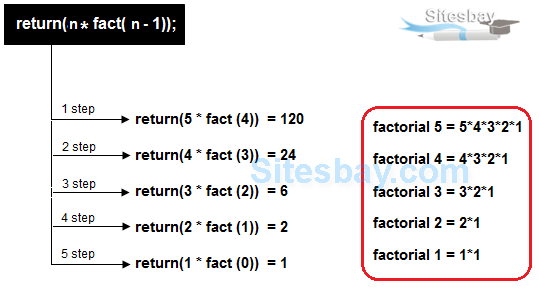# Recursion in C

## Recursive Function in C

When Function is call within same function is called Recursion. The function which call same function is called recursive function. In other word when a function call itself then that function is called Recursive function.

Recursive function are very useful to solve many mathematical problems like to calculate factorial of a number, generating Fibonacci series, etc.

• Function calling related information will be maintained by recursion.
• Stack evaluation will be take place by using recursion.
• In fix prefix, post-fix notation will be evaluated by using recursion.

• It is a very slow process due to stack overlapping.
• Recursive programs can create stack overflow.
• Recursive functions can create as loops.

## Example

```#include<stdio.h>
#include<conio.h>

void main()
{
int fact(int);
int i,f,num;
clrscr();
printf("Enter any number: ");
scanf("%d",&num);
f=fact(num);
printf("Factorial: %d",f);
getch();
}

int fact(int n)
{
if(a<0)
return(-1);
if(a==0)
return(1);
else
{
return(n*fact(n-1));
}
}
```

## Output

```Enter any number: 5
Factorial: 120
```## Example

```#include<stdio.h>
#include<conio.h>

void main()
{
int table(int,int);
int n,i;      // local variable
clrscr();
printf("Enter any num : ");
scanf("%d",&n);
for(i=1;i< =10;i++)
{
printf(" %d*%d= %d\n",n,i,table(n,i));
}
getch();
}
int table(n,i)
{
int t;
if(i==1)
{
return(n);
}
else
{
t=(table(n,i-1)+n);
return(t);
//return(table(n,i-1)+n);
}
}
```

## Output

```Enter any number: 5
5*1= 5
5*2= 10
5*3= 15
5*4= 20
5*5= 25
5*6= 30
5*7= 35
5*8= 40
5*9= 45
5*10= 50
```